# Quadratic equation + reason - math problems

#### Number of problems found: 48

• Trapezoid MOThe rectangular trapezoid ABCD with the right angle at point B, |AC| = 12, |CD| = 8, diagonals are perpendicular to each other. Calculate the perimeter and area of ​​the trapezoid.
• RightDetermine angles of the right triangle with the hypotenuse c and legs a, b, if: 3a +4b = 4.9c
• PoolIf water flows into the pool by two inlets, fill the whole for 19 hours. The first inlet filled pool 5 hour longer than the second. How long pool take to fill with two inlets separately?
• Pumps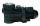The tank is filled with two pumps in 16 minutes. The first pump is filled in 30 minutes earlier than two one. How many minutes is filled with the first pump?
• Euler problem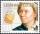Someone buys a 180 tolars towels. If it was for the same money of 3 more towels, it would be 3 tolars cheaper each. How many were towels?
• Secret number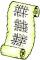Determine the secret number n, which reversed decrease by 16.4 if the number increase by 16.4.
• Party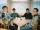At the party everyone clink with everyone. Together, they clink 406 times. How many people were at the party?
• Trolleybus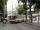Trolleybus line No. 206 measured 24 km. If the trolleybus goes faster by 5 km/h, the way there and back would is shorter by 33 minutes. Calculate the trolleybus speed and how much time it takes a return trip.

We apologize, but in this category are not a lot of examples.
Do you have an exciting math question or word problem that you can't solve? Ask a question or post a math problem, and we can try to solve it.

We will send a solution to your e-mail address. Solved examples are also published here. Please enter the e-mail correctly and check whether you don't have a full mailbox.

Looking for help with calculating roots of a quadratic equation? Quadratic Equations Problems. Reason - math problems.